# Thermal Dispersion Effects on Convection Heat Transfer in Porous Media with Viscous Dissipation

Thermal Dispersion Effects on Convection Heat Transfer in Porous Media with Viscous Dissipation

Ibraheem Nasser H. M. Duwairi*

Mechanical Engineering Department, Faculty of Engineering and Technology, the University of Jordan, 11942 Amman, Jordan

Corresponding Author Email:
duwairi@ju.edu.jo
Page:
207-212
|
DOI:
https://doi.org/10.18280/ijht.340208
|
Accepted:
|
Published:
30 June 2016
| Citation

OPEN ACCESS

Abstract:

The thermal dispersion effects on the Darcy–Forchheimer natural, mixed and forced convection heat transfer with viscous dissipation effects over an isothermal vertical flat plate in a fluid saturated porous media are examined numerically. The coefficient of thermal diffusivity has been assumed to be the sum of molecular diffusivity and the dispersion thermal diffusivity due to mechanical dispersion. The non-dimensional governing equations are solved by using finite difference method with a Crank Nicolson implicit numerical technique. The results show that in natural, mixed and forced convection heat transfer, when the modified Darcy number is increased the heat transfer rates are enhanced and when the modified Forchheimer number are increased the Nusselt numbers are decreased. The effect of both viscous dissipation and thermal dispersion was found to increase both velocities and temperatures inside boundary layer and to reduce heat transfer rates.

Keywords:

Thermal dispersion, Viscous dissipation, Porous media, Convection heat transfer.

1. Introduction

The thermal dispersion effect on convection heat transfer through porous media was studied by many investigators for several cases with various procedures: analytically, numerically and experimentally. Significant advances have been made in modeling fluid flow, heat, and mass transfer through a porous medium including the Brinkman–Forchheimer-extended of Darcy law or the generalized model.

Representative studies in this area may be found in the recent research [1-4]. The magnetic field and heat generation effects were studied numerically by boundary layer analysis for free convection heat and mass transfer along a vertical plate for a nano-fluid saturated porous medium in the presence of viscous dissipation effect by Ram Reddy et al. . The effects of variable viscosity and double dispersion on natural convection heat and mass transfer form a vertical cone in a saturated  porous medium with non-Newtonian fluid was studied by Kairi . The coupled heat and mass transfer by free convection in porous media had been presented for the case of vertical surface with linear temperature and concentration distribution by Rani and Rao . Morth at al.  studied the case of horizontal surface with linear temperature and concentration distribution, a boundary layer analysis for the mixed convection from a vertical plate embedded in a porous medium was presented. The effects of viscous dissipation on natural convection heat and mass transfer along a vertical plate embedded in saturated porous medium had been presented by MoorthY and Senthilvadivu . The effect of variable viscosity on unsteady mixed convection boundary layer flow over a vertical surface embedded in a porous medium were obtained by Hassanien et al. . The flow of an unsteady mixed-convection of fluid past an infinite vertical plate with time-dependent suction under the simultaneous effects of viscous dissipation was discussed by Ramreddy et al. . Viscous dissipation effects on Darcy-Forchheimer mixed convection in a fluid saturated porous media were examined by Hamad and Bashir . The MHD-mixed convection heat transfer problem was analyzed with viscous dissipation and Joule heating effects by Ibrahim et al. . Convective flow and heat transfer in a square porous cavity was investigated numerically by Mahdy and Chamkha . In this study, the thermal dispersion effects on the Darcy–Forchheimer natural, mixed and forced convection heat transfer with viscous dissipation effects over an isothermal vertical flat plate in a fluid saturated porous media will be examined numerically.

2. Mathematical Formulation

Attention will be concentrated on convection in a porous medium adjacent to a heated vertical flat plate, on which a thin thermal boundary layer is formed under the effect of externally forced and naturally heated stream flow over a vertical flat plate. As shown in following Figureure the governing equations that describe the problem under consideration can be written as:

## figure_1.png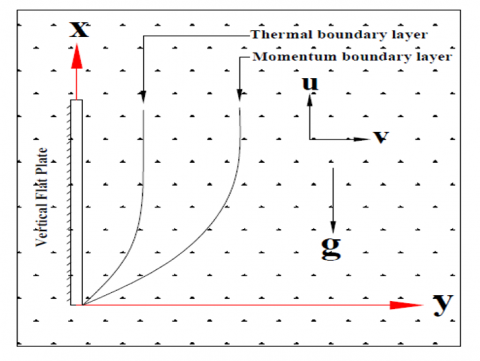Figure 0. Geometry plate in saturated porous media

The continuity equation:

$\frac{\partial \mathrm{u}}{\partial \mathrm{x}}+\frac{\partial \mathrm{v}}{\partial \mathrm{y}}=0$

The x-momentum equation:

$\frac{\partial \mathrm{u}}{\partial \mathrm{t}}+\frac{\mu \varepsilon}{\rho \mathrm{K}} \mathrm{u}+\frac{\mathrm{C}_{\mathrm{F}} \varepsilon^{2}}{\sqrt{\mathrm{K}}} \mathrm{u}^{2}=-\frac{1}{\rho} \frac{\partial \mathrm{P}}{\partial \mathrm{x}}+\mathrm{g}_{\mathrm{x}} \beta\left(\mathrm{T}-\mathrm{T}_{\infty}\right)$

The y-momentum equation:

$\frac{\partial \mathrm{v}}{\partial \mathrm{t}}+\frac{\mu \varepsilon}{\rho \mathrm{K}} \mathrm{v}+\frac{\mathrm{C}_{\mathrm{F}} \varepsilon^{2}}{\sqrt{\mathrm{K}}} \mathrm{v}^{2}=-\frac{1}{\rho} \frac{\partial \mathrm{P}}{\partial \mathrm{y}}+\mathrm{g}_{\mathrm{y}} \beta\left(\mathrm{T}-\mathrm{T}_{\infty}\right)$

The energy equation:

$\frac{\partial \mathrm{T}}{\partial \mathrm{t}}+\mathrm{u} \frac{\partial \mathrm{T}}{\partial \mathrm{x}}+\mathrm{v} \frac{\partial \mathrm{T}}{\partial \mathrm{y}}=\frac{\mathrm{k}}{\rho \mathrm{C}_{\mathrm{P}}}\left(\frac{\partial^{2} \mathrm{T}}{\partial \mathrm{x}^{2}}+\frac{\partial^{2} \mathrm{T}}{\partial \mathrm{y}^{2}}\right)+\frac{v}{\mathrm{KC}_{\mathrm{P}}} \mathrm{u}\left(\mathrm{u}+\frac{\mathrm{C}_{\mathrm{F}} \sqrt{\mathrm{K}}}{v} \mathrm{u}^{2}\right)$

With the following boundary conditions

$\begin{array}{ll}{\mathrm{y} \rightarrow \infty} & {\left(\mathrm{v}=0, \mathrm{u}=\mathrm{u}_{\infty} \& T=\mathrm{T}_{\infty}\right)} \\ {\mathrm{y} \rightarrow 0} & {\left(\mathrm{v}=0 \& T=\mathrm{T}_{\mathrm{w}}\right)}\end{array}$

Rewrite the y-momentum equation along the boundary layer edge ( $\mathrm{y} \rightarrow \infty$ ) utilizing the boundary conditions $\left(\mathrm{v}=0, \mathrm{u}=\mathrm{u}_{\infty} \quad \& \mathrm{T}=\mathrm{T}_{\infty}\right)$ .

To find $\left[-\frac{1}{\rho} \frac{\partial P}{\partial x}\right]$ . Then substitute it in the x-momentum equation to find the following equation.

$\frac{\mu \varepsilon}{\rho \mathrm{K}}\left(\mathrm{u}_{\infty}-\mathrm{u}\right)+\frac{\mathrm{C}_{\mathrm{F}} \varepsilon^{2}}{\sqrt{\mathrm{K}}}\left(\mathrm{u}_{\infty}^{2}-\mathrm{u}^{2}\right)+\mathrm{g}_{\mathrm{x}} \beta\left(\mathrm{T}-\mathrm{T}_{\infty}\right)=0$

The following dimensionless variables are defined:

$\mathrm{X}=\frac{\mathrm{x}}{\mathrm{L}}, \mathrm{Y}=\frac{\mathrm{y}}{\mathrm{L}}, \mathrm{U}=\frac{\mathrm{u}}{\mathrm{u}_{\infty}}, \mathrm{V}=\frac{\mathrm{v}}{\mathrm{u}_{\infty}}, \theta=\frac{\mathrm{T}-\mathrm{T}_{\infty}}{\mathrm{T}_{\mathrm{w}}-\mathrm{T}_{\infty}}$

$\tau=\frac{\mathrm{Ut}}{\mathrm{L}}, \mathrm{Gr}=\frac{\operatorname{Lg} \beta\left(\mathrm{T}_{\mathrm{w}}-\mathrm{T}_{\infty}\right)}{\mathrm{U}_{\infty}^{2}}, \mathrm{Da}^{*}=\frac{\mathrm{K} \rho \mathrm{u}_{\infty}}{\varepsilon \mathrm{L} \mu}$

$\mathrm{Fr}^{*}=\frac{\varepsilon^{2} \mathrm{C}_{\mathrm{F}} \mathrm{L}}{\sqrt{\mathrm{K}}}, \mathrm{Pe}=\frac{\mathrm{L} \mathrm{u}_{\infty}}{\alpha}, \mathrm{Ec}=\frac{\mathrm{U}_{\infty}^{2}}{\mathrm{C}_{\mathrm{P}}\left(\mathrm{T}_{\mathrm{w}}-\mathrm{T}_{\infty}\right)}, \mathrm{T}_{\mathrm{d}}=\frac{\gamma \mathrm{d}}{\mathrm{L}}$

where Da* is the modified Darcy number, Fr* is the modified Forchhimer number, Pe is Peclet Number, Ec is Eckert number and Td is thermal dispersion number. So the continuity, the x-momentum and energy equations can be rewritten as:

$\frac{\partial \mathrm{U}}{\partial \mathrm{X}}+\frac{\partial \mathrm{V}}{\partial \mathrm{Y}}=0$

• For natural convection heat transfer

$\mathrm{U}=\frac{-1}{2 \mathrm{Da}^{*} \mathrm{Fr}^{*}}+\left[\left(\frac{1}{2 \mathrm{Da}^{*} \mathrm{Fr}^{*}}\right)^{2}+\frac{\mathrm{Gr} \theta}{\mathrm{Fr}^{*}}\right]^{\frac{1}{2}}$

where $\mathrm{u}_{\infty}$  is reference velocity defined as:

$\mathrm{u}_{\infty}=-\frac{v}{2 \epsilon \mathrm{C}_{\mathrm{F}} \sqrt{\mathrm{K}}}+\left[\left(\frac{v}{2 \epsilon \mathrm{C}_{\mathrm{F}} \sqrt{\mathrm{K}}}\right)^{2}+\frac{\mathrm{g} \beta \sqrt{\mathrm{K}}\left(\mathrm{T}_{\mathrm{w}}-\mathrm{T}_{\infty}\right)}{\epsilon \mathrm{C}_{\mathrm{F}}}\right]^{0.5}$ .

• For mixed and forced convection heat transfer.

$\mathrm{U}=\frac{-1}{2 \mathrm{Da}^{*} \mathrm{Fr}^{*}}+\left[\left(\frac{1}{2 \mathrm{Da}^{*} \mathrm{Fr}^{*}}+1\right)^{2}+\frac{\mathrm{Gr} \theta}{\mathrm{Fr}^{*}}\right]^{1 / 2}$

Energy equation:

$\frac{\partial \theta}{\partial \tau}+\mathrm{U} \frac{\partial \theta}{\partial \mathrm{X}}+\mathrm{V} \frac{\partial \theta}{\partial \mathrm{Y}}=\left(\frac{1}{\mathrm{Pe}}+\mathrm{T}_{\mathrm{d}}\right) \frac{\partial^{2} \theta}{\partial \mathrm{Y}^{2}} \quad+\operatorname{Ec} \mathrm{U}\left(\frac{1}{\mathrm{Da}^{*}} \mathrm{U}+\mathrm{Fr}^{*} \mathrm{U}^{2}\right)$

The effective thermal diffusivity (ae) may be expressed as $\alpha_{\mathrm{e}}=\alpha+\alpha_{\mathrm{d}}$ . The molecular thermal diffusivity is assumed be constant and the dispersion thermal diffusivity is written as $\alpha_{\mathrm{d}}=\gamma \mathrm{d} \mathrm{u}$ .

3. Numerical Solution

Crank Nicolson implicit numerical technique will be used to solve the continuity, momentum and energy coupled equations. The dimensionless temperature and velocity derivative respect to x, y and t will be written as:

The continuity equation:

$\mathrm{V}_{\mathrm{i}, \mathrm{j}}^{\mathrm{n}+1}=-\frac{\Delta \mathrm{y}}{\Delta \mathrm{x}}\left[\mathrm{U}_{\mathrm{i}, \mathrm{j}}^{\mathrm{n}+1}-\mathrm{U}_{\mathrm{i}, \mathrm{j}}^{\mathrm{n}}\right]+\mathrm{V}_{\mathrm{i}-1, \mathrm{j}}^{\mathrm{n}+1}$

The momentum Equation in x-direction:

$\mathrm{U}_{\mathrm{i}, \mathrm{j}}^{\mathrm{n}+1}=\frac{-1}{2 \mathrm{Da}^{*} \mathrm{Fr}^{*}}+\left[\left(\frac{1}{2 \mathrm{Da}^{*} \mathrm{Fr}^{*}}+1\right)^{2}+\frac{\mathrm{Gr} \theta_{\mathrm{j}, \mathrm{i}}^{\mathrm{n}+1}}{\mathrm{Fr}^{*}}\right]^{0.5}$

The Energy Equation:

$\frac{\theta_{\mathrm{j}, \mathrm{i}}^{\mathrm{n}+1}-\theta_{\mathrm{j}, \mathrm{i}}^{\mathrm{n}}}{\Delta \tau}=-\mathrm{U}_{\mathrm{i}, \mathrm{j}}^{\mathrm{n}}\left[\frac{\theta_{\mathrm{j}, \mathrm{i}+1}^{\mathrm{n}}-\theta_{\mathrm{j}, \mathrm{i}}}{\Delta \mathrm{x}}\right]$

$-+\frac{V_{i, j}^{n}}{2}\left[\frac{\theta_{j+1, i} n^{n+1}-\theta_{j, i}^{n+1}+\theta_{j+1, i}^{n}-\theta_{j, i}^{n}}{\Delta y}\right]$

$+\left(\frac{1}{2 \mathrm{Pe}}+\frac{\mathrm{T}_{\mathrm{d}} \mathrm{U}_{\mathrm{j}, \mathrm{i}}^{\mathrm{n}}}{2}\right) \ldots$

$\left[\frac{\theta_{\mathrm{j}+1, \mathrm{i}}^{\mathrm{n}+1}-2 \theta_{\mathrm{j}, \mathrm{i}}^{\mathrm{n}+1}+\theta_{\mathrm{j}+1, \mathrm{i}}^{\mathrm{n}+1}+\theta_{\mathrm{j}+1, \mathrm{i}}^{\mathrm{n}}-2 \theta_{\mathrm{j}, \mathrm{i}}^{\mathrm{n}}+\theta_{\mathrm{j}+1, \mathrm{i}}^{\mathrm{n}}}{(\Delta \mathrm{y})^{2}}\right]$

$+\operatorname{Ec} U_{\mathrm{j}, \mathrm{i}}^{\mathrm{n}}\left[\frac{1}{\mathrm{Da}^{*}} \mathrm{U}_{\mathrm{j}, \mathrm{i}}^{\mathrm{n}}+\mathrm{Da}^{*} \mathrm{Fr}^{*}\left(\mathrm{U}_{\mathrm{j}, \mathrm{i}} \mathrm{U}_{\mathrm{j}, \mathrm{i}}\right)^{\mathrm{n}}\right]$

After subtitling the finite difference approximation in energy equation, it has the following form which includes three unknown at present time:

$A_{j} \theta_{j+1, i}^{n+1}+B_{j} \theta_{j, i}^{n+1}+C_{j} \theta_{j-1, i}^{n+1}=D_{j}$

$A_{j}=1-\frac{V_{j, i}^{n} \Delta \tau}{2(\Delta y)}+\frac{\Delta \tau}{\operatorname{Pe}(\Delta y)^{2}}+\frac{T_{d} U_{j, i}^{n} \Delta \tau}{(\Delta y)^{2}}$

$\mathrm{B}_{\mathrm{j}}=\frac{\mathrm{V}_{\mathrm{j}, \mathrm{i}}^{\mathrm{n}} \Delta \tau}{2(\Delta \mathrm{y})}+\frac{\Delta \tau}{2 \operatorname{Pe}(\Delta \mathrm{y})^{2}}+\frac{\mathrm{T}_{\mathrm{d}} \mathrm{U}_{\mathrm{j}, \mathrm{i}}^{\mathrm{n}} \Delta \tau}{2(\Delta \mathrm{y})^{2}}$

$\mathrm{C}_{\mathrm{j}}=-\frac{\Delta \tau}{2 \operatorname{Pe}(\Delta \mathrm{y})^{2}}-\frac{\mathrm{T}_{\mathrm{d}} \mathrm{U}_{\mathrm{j}, \mathrm{i}}^{\mathrm{n}} \Delta \tau}{2(\Delta \mathrm{y})^{2}}$

$D_{j}=\theta_{i, j}^{n}+\Delta \tau\left[-U_{i, j}^{n} \frac{\theta_{j, i}^{n}-\theta_{j, i-1}^{n}}{\Delta x}\right.$$-\frac{\mathrm{V}_{\mathrm{i}, \mathrm{j}}^{\mathrm{n}}}{2} \frac{\theta_{\mathrm{j}+1, \mathrm{i}}^{\mathrm{n}}-\theta_{\mathrm{j}, \mathrm{i}}^{\mathrm{n}}}{\Delta \mathrm{y}} ]$$+\left(\frac{1}{2 \mathrm{Pe}}+\frac{\mathrm{T}_{\mathrm{d}} \mathrm{U}_{\mathrm{j}, \mathrm{i}}^{\mathrm{n}}}{2}\right)\left[\frac{\theta_{\mathrm{j}+1, \mathrm{i}}^{\mathrm{n}}-2 \theta_{\mathrm{j}, \mathrm{i}}^{\mathrm{n}}+\theta_{\mathrm{j}-1, \mathrm{i}}^{\mathrm{n}}}{(\Delta \mathrm{y})^{2}}\right]$$+\operatorname{Ec} \mathrm{U}_{\mathrm{j}, \mathrm{i}}^{\mathrm{n}}\left[\frac{1}{\mathrm{Da}^{*}} \mathrm{U}_{\mathrm{j}, \mathrm{i}}^{\mathrm{n}}+\mathrm{Fr}^{*}\left(\mathrm{U}_{\mathrm{j}, \mathrm{i}} \cdot \mathrm{U}_{\mathrm{j}, \mathrm{i}}\right)^{\mathrm{n}}\right]$

The boundary condition defined the dimensionless temperature at y=0 and $\mathrm{y}=\infty$ are equal 1 and 0 respectively. At every i -line j will be equal 1, 2, 3… n-1, n. a set of n equations in the n unknown value of  $\theta$ is obtained.

The above equation are solved to find the dimensionless temperature at certain i-line after that the dimensionless temperature will be founded at next i-line until all them will be calculated at every i and j at certain time until reaching steady state.

The local Nusselt number is given by:

$\mathrm{N}_{\mathrm{u}}=\left[\frac{\theta_{\mathrm{j}, \mathrm{i}}^{\mathrm{n}}-\theta_{\mathrm{j}-1, \mathrm{i}}^{\mathrm{n}}}{\Delta \mathrm{y}}\right]_{\mathrm{y}=0}$

4. Results and Conclusion

The velocity, temperature and local Nusselt number have been computed by using finite difference method (Crank Nicolson Technique). The numerical calculations are carried out for the effect of the modified Darcy number, modified Forchhimer number, viscous dissipation and thermal dispersion.

The velocity and temperature profiles and local Nusselt numbers for different values of modified Darcy number [1, 10, 100] and Fr*=0.1, Pe=7, Ec=0.01, Td=0.1, Gr=1 for natural, mixed and forced convection are drawn in Figures (1, 2, 3) respectively. It is clear that the increasing of modified Darcy number increased velocities and decreased thermal boundary layer thickness which results in higher coefficients of heat transfer. The effect of Forcheimer’s number [0.05, 0.1, 0.5] and Da*=10, Pe=7, Ec=0.01, Td=0.1, on natural, mixed and forced convection are drawn if Figures (4, 5, 6). It is found that the increasing of Forecheimr’s number decreased velocities and broadens temperature distribution and consequently decreased coefficient of heat transfer and Nusselt numbers.

The viscous dissipation effects are presented in Figures (7, 8, 9) for Ec=0, 0.1, 0.2 and Da*=10, Fr=0.1, Pe=7, Td=0.1, Gr=1. It is found that the increasing of viscous dissipation parameter or Eckert number had increased velocities inside boundary layers and heated the fluid which consequently decreased heat transfer rates; this is due to the important role played by viscosity in raising temperatures of flowing fluids.

The effects of thermal dispersion are shown if Figures (11, 12, 13) for different values of Td=0, 0.1, 0.2 1 and Da*=0.1, Fr*=0.1, Pe=7, Ec=0.01. It is clear that the effect of thermal dispersion is to increase both velocities and temperatures which consequently results in smaller heat transfer rates.

• Modified Darcy Number

## figure_1.png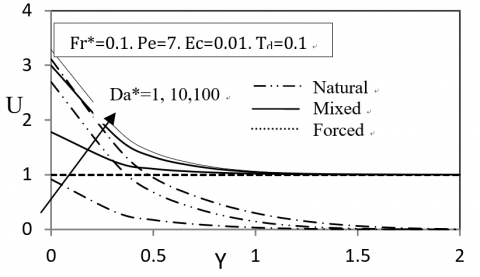Figure 1. Dimensionless velocity Profiles for different values of modified Darcy number

## figure_2.png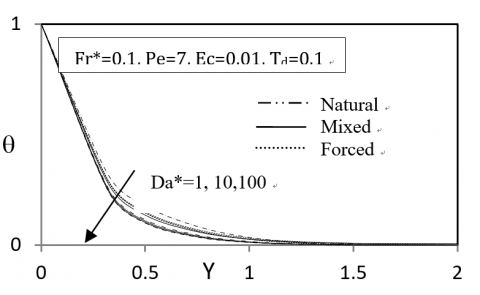Figure 2. Dimensionless temperature Profiles for different values of modified Darcy number

## figure_3.png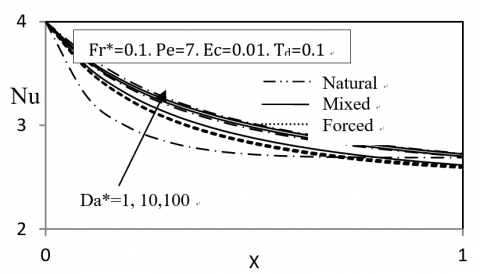Figure 3. Local Nusselt number as function for different values of modified Darcy number

• Modified Forchhimer Number

## figure_4.png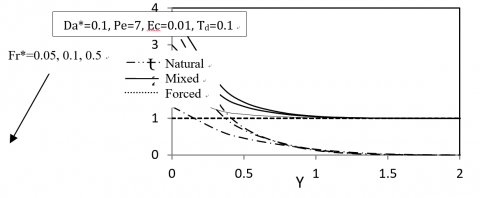Figure 4. Dimensionless velocity Profiles for different values of modified Forchhimer number

## figure_5.png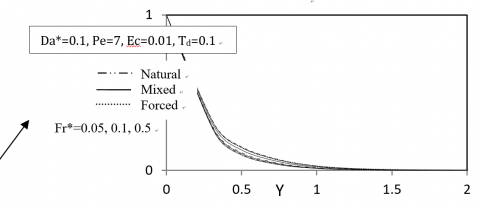Figure 5. Dimensionless temperature Profiles for different values of modified Forchhimer number

## figure_6.png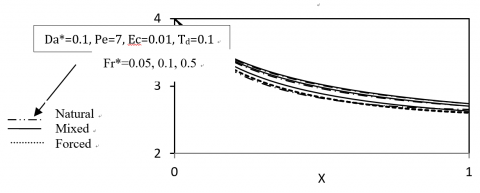Figure 6. Local Nusselt number for different modified values of Forchhimer number

• Viscous dissipation

## figure_7.png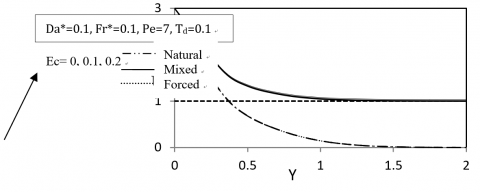Figure 7. Dimensionless velocity Profiles for different values of viscous dissipation parameter

## figure_8.png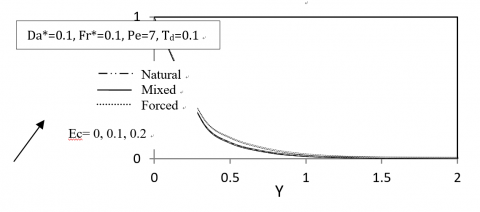Figure 8. Dimensionless temperature Profiles for different values of viscous dissipation parameter

## figure_9.png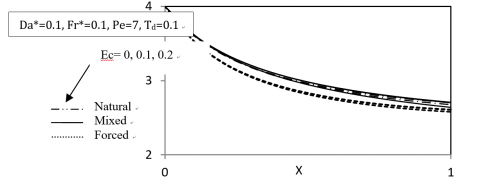Figure 9. Local Nusselt number for different viscous dissipation

• Thermal dispersion

## figure_10.png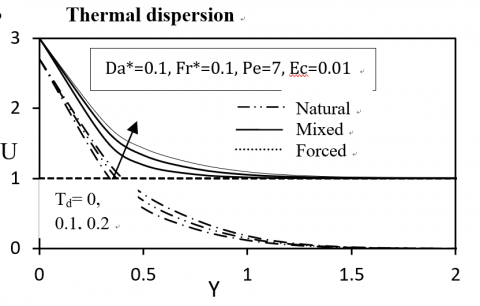Figure 10. Dimensionless velocity Profiles for different values of thermal dispersion parameter

## figure_11.png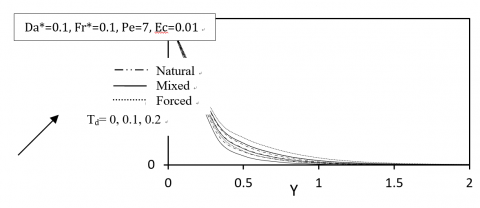Figure 11. Dimensionless temperature Profiles for different values of thermal dispersion parameter

## figure_12.png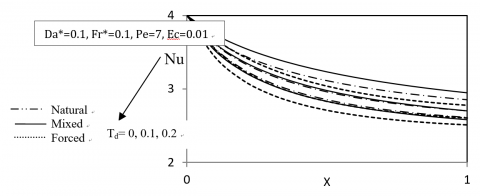Figure 12. Local Nusselt number for different values of thermal dispersion parameter

5. Conclusions

The thermal dispersion effects on the Darcy–Forchheimer natural, mixed and forced convection heat transfer with viscous dissipation effects over an isothermal vertical flat plate in a fluid saturated porous media are examined numerically. The non-dimensionalized conservation equations have been solved using the finite difference method whereas the coefficient of thermal diffusivity has been assumed to be the sum of molecular diffusivity and the dispersion thermal diffusivity due to mechanical dispersion. The major findings of the study are summarized as follows:

1. When the modified Darcy number is increased, the velocities inside boundary layers are increased and the temperatures are decreased which results in higher coefficients of heat transfer.
2. When the modified Forcheimer numbers are increased, the velocities are decreased and the temperatures are increased which decreased local Nusselt numbers.
3. When the Eckert numbers or viscous dissipation parameter are increased, both the velocities and temperatures are increased but the coefficients of heat transfer are decreased
4. When the thermal dispersion parameter is increased, both the velocities and temperatures are increased and local Nusselt numbers are decreased; this is due to excessive heating of fluid layers.
Nomenclature
 CP Specific heat (J/Kg.K). CF Friction Coefficient. g Gravitational acceleration (m/s2). h Convection heat transfer coefficient. (W/m2.k). K Permeability (m2). Nu Local Nusselt number. q' Heat transfer rate per unit length (W/m). $\varepsilon$ The porosity of the porous medium. u, v Velocity components in x and y directions. (m/s). U, V Dimensionless velocity components in x and y directions. T Temperature (K). $\theta$ Dimensionless temperature. P Pressure (Pa). x Axial coordinate along the plate (m). X Dimensionless axial coordinate along the plate. y Normal coordinate normal to the plate (m). L Length of vertical plate (m). Y Dimensionless vertical coordinate to the plate. t Time (s). $\tau$ Dimensionless time. Gr Grashof Number. Da* Modified Darcy Number. Fr* Modified Forchhimer Number. Pe Peclet number. Ec Eckert Number. Td Thermal Dispresion Effect Number. Greek letters $\alpha_{m}$ Effective thermal diffusivity of the porous media $\alpha$ Molecular thermal diffusivity. $\alpha_{\mathrm{d}}$ Dispersion thermal diffusivity. $\gamma$ Coefficient of mechanical dispersion. d Pore diameter. $\mu$ Dynamic viscosity $v$ Kinematic viscosity
References

 H. M. Duwairi, “Radiation effects on mixed convection over a nonisothermal cylinder and sphere in a porous media,” Journal of Porous Media, vol. 9, no. 3, pp. 252-259, 2006. DOI: 10.1615/JPorMedia.v9.i3.60.

 T. K Aldoss, M. A Jarrah, H. M Duwairi, “Wall effect on mixed convection from horizontal surfaces with a variable surface heat flux,” The Canadian Journal of Chemical Engineering, vol. 72, no.1 pp. 35-24, 1994. DOI: 10.1002/cjce.5450720106.

 H. M Duwairi, T. K Aldoss, M. A Jarrah, “Nonsimilarity solutions for non-Darcy mixed convection from horizontal surfaces in a porous medium,” Heat and Mass Transfer, vol. 33 no. 1-2, pp. 149-156, 1997. DOI: 10.1007/s002310050172.

 H. M. Duwairi, Y.  Al-Kablawi, “MHD-conjugate mixed convection heat transfer over a vertical hollow cylinder embedded in a porous medium,” International Journal of Heat and Technology, vol. 24, no.1, pp. 123-128, 2006.

 Ch. RamReddy, P.V.S.N. Murthy, A. J. Chamkha and A. M. Rashad. “Influence of viscous dissipation on free convection in a non-Darcy porous medium saturated with nanofluid in the presence of magnetic field,” The Open Transport Phenomena Journal, vol. 5, pp. 20-29, 2013. DOI: 10.2174/1877729501305010020.

 Rishi Raj KAIRI, “Viscosity and dispersion effects on natural convection from a vertical cone in a non-Newtonian fluid saturated porous medium.” Thermal Science, vol. 15, no. 2, pp. 307-316, 2011.

 Raja Rani. T and C.N.B. Rao, “Effects of variable fluid properties on free convection flows and heat transfer at an isothermal vertical plate embedded in a porous medium in the presence of magnetic field and radiation,” International Journal of Advanced Research in Computer Science and Software Engineering, vol. 3, no. 5, May 2013.

 M.B.K. MoorthY and K. Senthilvadivu, “Effect of variable viscosity on convective heat and mass transfer by natural convection from vertical surface in porous medium,” Wseas Transactions on Mathematics, vol. 11, no. 9, September 2012.

 M.B.K. MoorthY and K. Senthilvadiv, “Effect of variable viscosity on convective heat and mass transfer by natural convection from horizontal surface in porous medium,” Wseas Transactions on Mathematics, vol. 10, no. 6, June 2011.

 I. A. Hassanien, A. Y. Bakier and R. S. R. Gorla “Effects of thermal dispersion and stratification on non-darcy mixed convection from a vertical plate in a porous medium,” International Journal of Heat and Mass Transfer, vol. 34, no. 2-3, pp. 209-212, 1998.

 Ch. Ramreddy, P.V.S.N. Murthy, A. J. Chamkha  and A.M. Rashad, “Influence of viscous dissipation on free convection in a non-Darcy porous medium saturated with nanofluid in the presence of magnetic field,” The Open Transport Phenomena Journal, vol. 5, pp. 20-29, 2013.

 M.A.A. Hamad and Muatazz Abdolhadi Bashir, “Similarity Solutions of the Effect of Variable Viscosity on Unsteady Mixed Convection Boundary Layer Flow over a Vertical Surface Embedded in a Porous Medium via HAMAD Formulations,” World Applied Sciences Journal, vol. 12, no. 4, pp. 519-530, 2011.

 F. S. Ibrahim, A. M. Elaiw and A. A. Bakr. “Influence of viscous dissipation and radiation on unsteady MHD Mixed convection flow of micropolar fluids,” Applied Mathematics & Information Sciences, vol. 2, no. 2, pp. 143–162, 2008.

 A. Mahdy, A. J. Chamkha, “Chemical reaction and viscous dissipation effects on Darcy-Forchheimer mixed convection in a fluid saturated porous media,” International Journal of Numerical Methods for Heat & Fluid Flow, vol. 20, no. 8, 2010. DOI: 10.1108/09615531011081441.